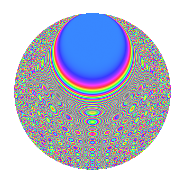# Properties

 Label 864.2.bnLevel 864 Weight 2 Character orbit bn Rep. character $$\chi_{864}(35,\cdot)$$ Character field $$\Q(\zeta_{24})$$ Dimension 368 Newform subspaces 1 Sturm bound 288 Trace bound 0

# Related objects

## Defining parameters

 Level: $$N$$ = $$864 = 2^{5} \cdot 3^{3}$$ Weight: $$k$$ = $$2$$ Character orbit: $$[\chi]$$ = 864.bn (of order $$24$$ and degree $$8$$) Character conductor: $$\operatorname{cond}(\chi)$$ = $$288$$ Character field: $$\Q(\zeta_{24})$$ Newform subspaces: $$1$$ Sturm bound: $$288$$ Trace bound: $$0$$

## Dimensions

The following table gives the dimensions of various subspaces of $$M_{2}(864, [\chi])$$.

Total New Old
Modular forms 1200 400 800
Cusp forms 1104 368 736
Eisenstein series 96 32 64

## Trace form

 $$368q + 12q^{2} - 4q^{4} + 12q^{5} - 4q^{7} + O(q^{10})$$ $$368q + 12q^{2} - 4q^{4} + 12q^{5} - 4q^{7} - 16q^{10} + 12q^{11} - 4q^{13} + 12q^{14} - 4q^{16} - 16q^{19} + 12q^{20} - 4q^{22} + 12q^{23} - 4q^{25} - 16q^{28} + 12q^{29} + 12q^{32} - 12q^{34} - 16q^{37} + 12q^{38} - 4q^{40} + 12q^{41} - 4q^{43} - 16q^{46} + 24q^{47} + 168q^{50} - 4q^{52} - 16q^{55} + 12q^{56} + 32q^{58} + 12q^{59} - 4q^{61} - 16q^{64} + 24q^{65} - 4q^{67} + 60q^{68} - 4q^{70} - 16q^{73} + 12q^{74} - 28q^{76} + 12q^{77} - 8q^{79} - 16q^{82} + 132q^{83} - 24q^{85} + 12q^{86} - 4q^{88} - 16q^{91} - 216q^{92} - 20q^{94} - 8q^{97} + O(q^{100})$$

## Decomposition of $$S_{2}^{\mathrm{new}}(864, [\chi])$$ into newform subspaces

Label Dim. $$A$$ Field CM Traces $q$-expansion
$$a_2$$ $$a_3$$ $$a_5$$ $$a_7$$
864.2.bn.a $$368$$ $$6.899$$ None $$12$$ $$0$$ $$12$$ $$-4$$

## Decomposition of $$S_{2}^{\mathrm{old}}(864, [\chi])$$ into lower level spaces

$$S_{2}^{\mathrm{old}}(864, [\chi]) \cong$$ $$S_{2}^{\mathrm{new}}(288, [\chi])$$$$^{\oplus 2}$$

## Hecke Characteristic Polynomials

There are no characteristic polynomials of Hecke operators in the database